Learning Library

Probability Practice

Click on a worksheet in the set below to see more info or download the PDF.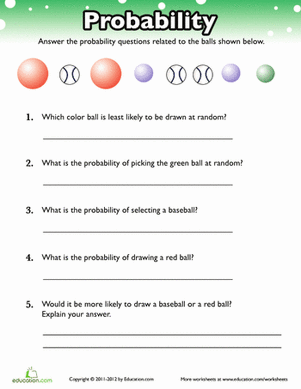Probability Practice

Get some practice with probability! With this exercise, your child will practice using fractions to express probability of different outcomes.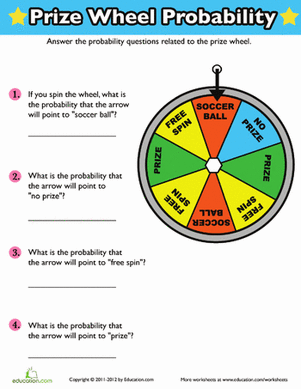Probability Prize Wheel

Give your child some practice with probability! With this exercise, he'll determine how likely each outcome of the prize wheel is.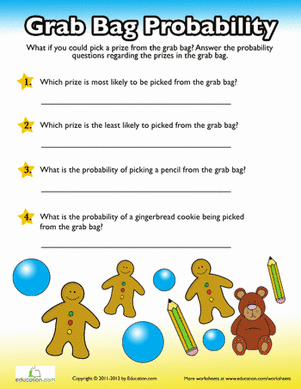Probability Grab Bag

Picking a random prize isn't just about luck, it involves math too! With this worksheet, your child will practice determining probability with the grab bag.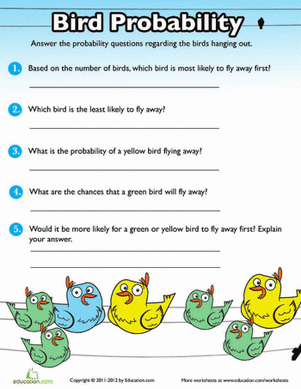Probability Practice: Birds

Practice probability and fractions with some feathery friends! In this exercise, your child will determine the likelihood of certain birds flying away.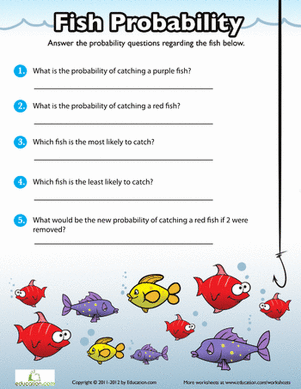Probability Practice: Fish

What kind of fish would you like to catch? Help your child master the concept of probability with this fun, visual exercise!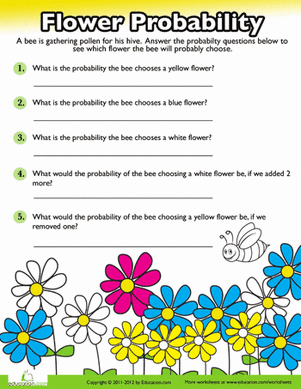Probability Practice: Flowers

Can you guess which flower the bee will choose? With this exercise your child will learn how to determine the likelihood of the bee choosing a certain flower.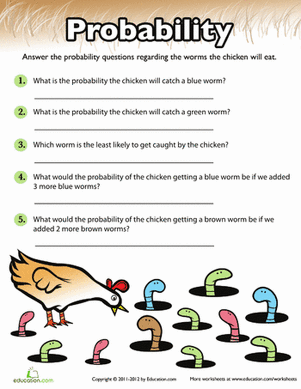Probability Practice: Worms

The early bird gets the worm! But which one will he choose? Have your child practice determining probability with a fun, visual exercise.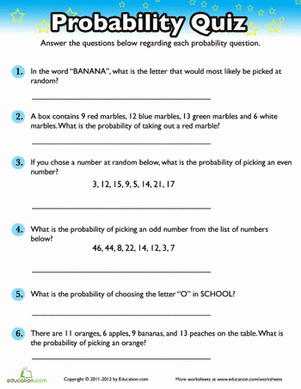Probability Quiz

Here's a great opportunity for your child to practice probability! He'll solve word problems about the probability of choosing an item from a group.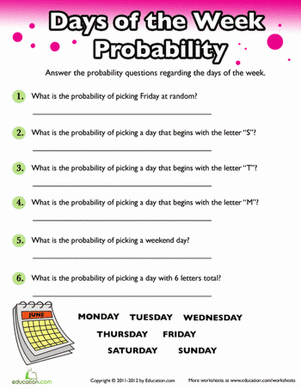Probability: Days of the Week

Practice probability with the days of the week! Your child will learn to find the probability of picking a random day on the calendar.

Want to download this whole set as a single PDF?

Create new collection

0

New Collection>

0 items

What could we do to improve Education.com?

Please note: Use the Contact Us link at the bottom of our website for account-specific questions or issues.

What would make you love Education.com?